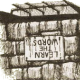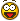# Point inside closed polyline

## Recommended PostsHi,

I have problem to find any point inside closed polyline.

The point can't be on edge this polyline.

I found example:

http://docs.autodesk.com/ACDMAC/2011/ENU/ObjectARX%20Reference/index.html?frmname=topic&frmfile=AcString.html

but this is for ARX application and I need for Lisp.

- figure on top

I have tried with triangles or intersection but without result...

Have someone have any idea?

##### Share on other sitesWhat about using the boundary command to test if the pl created from the point matches at least part of the original polyline?

##### Share on other sitesHere is some code based on a function written by gile:

```[color=GREEN];;----------------------=={ Inside-p }==----------------------;;[/color]
[color=GREEN];;                                                            ;;[/color]
[color=GREEN];;  Predicate function to determine whether a point lies      ;;[/color]
[color=GREEN];;  inside a supplied LWPolyline.                             ;;[/color]
[color=GREEN];;------------------------------------------------------------;;[/color]
[color=GREEN];;  Author: Lee Mac - www.lee-mac.com                         ;;[/color]
[color=GREEN];;  Using some code by gile (as marked below), thanks gile.   ;;[/color]
[color=GREEN];;------------------------------------------------------------;;[/color]
[color=GREEN];;  Arguments:                                                ;;[/color]
[color=GREEN];;  pt  - 3D WCS point to test                                ;;[/color]
[color=GREEN];;  ent - LWPolyline Entity against which to test point       ;;[/color]
[color=GREEN];;------------------------------------------------------------;;[/color]
[color=GREEN];;  Returns:  T if supplied point lies inside supplied LWPoly ;;[/color]
[color=GREEN];;------------------------------------------------------------;;[/color]

([color=BLUE]defun[/color] LM:Inside-p ( pt ent [color=BLUE]/[/color] _GroupByNum lst nrm obj tmp )

([color=BLUE]defun[/color] _GroupByNum ( l n [color=BLUE]/[/color] r)
([color=BLUE]if[/color] l
([color=BLUE]cons[/color]
([color=BLUE]reverse[/color] ([color=BLUE]repeat[/color] n ([color=BLUE]setq[/color] r ([color=BLUE]cons[/color] ([color=BLUE]car[/color] l) r) l ([color=BLUE]cdr[/color] l)) r))
(_GroupByNum l n)
)
)
)

([color=BLUE]if[/color] ([color=BLUE]=[/color] ([color=BLUE]type[/color] ent) 'VLA-OBJECT)
([color=BLUE]setq[/color] obj ent ent ([color=BLUE]vlax-vla-object->ename[/color] ent))
([color=BLUE]setq[/color] obj ([color=BLUE]vlax-ename->vla-object[/color] ent))
)

([color=BLUE]setq[/color] lst
(_GroupByNum
([color=BLUE]vlax-invoke[/color]
([color=BLUE]setq[/color] tmp
([color=BLUE]vlax-ename->vla-object[/color]
([color=BLUE]entmakex[/color]
([color=BLUE]list[/color]
([color=BLUE]cons[/color] 0 [color=MAROON]"RAY"[/color])
([color=BLUE]cons[/color] 100 [color=MAROON]"AcDbEntity"[/color])
([color=BLUE]cons[/color] 100 [color=MAROON]"AcDbRay"[/color])
([color=BLUE]cons[/color] 10 pt)
([color=BLUE]cons[/color] 11 ([color=BLUE]trans[/color] '(1. 0. 0.) ent 0))
)
)
)
)
'IntersectWith obj [color=BLUE]acextendnone[/color]
)
3
)
)
([color=BLUE]vla-delete[/color] tmp)
([color=BLUE]setq[/color] nrm ([color=BLUE]cdr[/color] ([color=BLUE]assoc[/color] 210 ([color=BLUE]entget[/color] ent))))

[color=GREEN];; gile:[/color]
([color=BLUE]and[/color] lst ([color=BLUE]not[/color] ([color=BLUE]vlax-curve-getparamatpoint[/color] ent pt))
([color=BLUE]=[/color] 1
([color=BLUE]rem[/color]
([color=BLUE]length[/color]
([color=BLUE]vl-remove-if[/color]
([color=BLUE]function[/color]
([color=BLUE]lambda[/color] ( p [color=BLUE]/[/color] pa p- p+ p0 s1 s2 ) ([color=BLUE]setq[/color] pa ([color=BLUE]vlax-curve-getparamatpoint[/color] ent p))
([color=BLUE]or[/color]
([color=BLUE]and[/color] ([color=BLUE]equal[/color] ([color=BLUE]fix[/color] ([color=BLUE]+[/color] pa ([color=BLUE]if[/color] ([color=BLUE]minusp[/color] pa) -0.5 0.5))) pa 1e-
([color=BLUE]setq[/color] p-
([color=BLUE]cond[/color]
( ([color=BLUE]setq[/color] p- ([color=BLUE]vlax-curve-getPointatParam[/color] ent ([color=BLUE]-[/color] pa 1e-))
([color=BLUE]trans[/color] p- 0 nrm)
)
( ([color=BLUE]trans[/color] ([color=BLUE]vlax-curve-getPointatParam[/color] ent ([color=BLUE]-[/color] ([color=BLUE]vlax-curve-getEndParam[/color] ent) 1e-) 0 nrm) )
)
)
([color=BLUE]setq[/color] p+
([color=BLUE]cond[/color]
( ([color=BLUE]setq[/color] p+ ([color=BLUE]vlax-curve-getPointatParam[/color] ent ([color=BLUE]+[/color] pa 1e-))
([color=BLUE]trans[/color] p+ 0 nrm)
)
( ([color=BLUE]trans[/color] ([color=BLUE]vlax-curve-getPointatParam[/color] ent ([color=BLUE]+[/color] ([color=BLUE]vlax-curve-getStartParam[/color] ent) 1e-) 0 nrm) )
)
)
([color=BLUE]setq[/color] p0 ([color=BLUE]trans[/color] pt 0 nrm))
([color=BLUE]<=[/color] 0 ([color=BLUE]*[/color] ([color=BLUE]sin[/color] ([color=BLUE]angle[/color] p0 p-)) ([color=BLUE]sin[/color] ([color=BLUE]angle[/color] p0 p+)))) [color=darkgreen];; LM Mod[/color]
)
([color=BLUE]and[/color]
([color=BLUE]/=[/color] 0. ([color=BLUE]vla-getBulge[/color] obj ([color=BLUE]fix[/color] pa)))
([color=BLUE]equal[/color] '(0. 0.) ([color=BLUE]cdr[/color] ([color=BLUE]trans[/color] ([color=BLUE]vlax-curve-getFirstDeriv[/color] ent pa) 0 nrm)) 1e-9)
)
)
)
)
lst
)
)
2
)
)
)
)```

Example test function:

```([color=BLUE]defun[/color] c:test ( [color=BLUE]/[/color] ss i lst pt ent )
([color=BLUE]if[/color]
([color=BLUE]and[/color] ([color=BLUE]setq[/color] ss ([color=BLUE]ssget[/color] [color=MAROON]"_X"[/color] '((0 . [color=MAROON]"LWPOLYLINE"[/color]))))
([color=BLUE]repeat[/color] ([color=BLUE]setq[/color] i ([color=BLUE]sslength[/color] ss))
([color=BLUE]setq[/color] lst ([color=BLUE]cons[/color] ([color=BLUE]ssname[/color] ss ([color=BLUE]setq[/color] i ([color=BLUE]1-[/color] i))) lst))
)
([color=BLUE]setq[/color] pt ([color=BLUE]getpoint[/color] [color=MAROON]"\nPick Point: "[/color]))
)
([color=BLUE]if[/color]
([color=BLUE]setq[/color] ent
([color=BLUE]car[/color]
([color=BLUE]vl-member-if[/color]
([color=BLUE]function[/color]
([color=BLUE]lambda[/color] ( x ) (LM:Inside-p ([color=BLUE]trans[/color] pt 1 0) x))
)
lst
)
)
)
([color=BLUE]vla-put-color[/color] ([color=BLUE]vlax-ename->vla-object[/color] ent) [color=BLUE]acRed[/color])
)
)
([color=BLUE]princ[/color])
)```

##### Share on other sitesObviously this will only work with a closed LWPolyline, but wouldn't using an XLine be a lot less legwork, or am I missing something?

```[color=yellow](defun _isPointInside (polygon point / temp test)
(if (vlax-curve-isClosed polygon)
(progn (setq test (eq (length (vlax-invoke
(setq temp (vlax-ename->vla-object
(entmakex (list '(0 . "XLINE")
'(100 . "AcDbEntity")
'(100 . "AcDbXline")
(cons 10 point)
(cons 11 (trans '(1. 0. 0.) polygon 0))
)
)
)
)
'IntersectWith
(vlax-ename->vla-object polygon)
acExtendNone
)
)
6
)
)
(vla-delete temp)
test
)
)
)[/color]```

Nevermind, I didn't think about if the polygon was irregularly shaped. My code is garbage, but I'll leave it there to show my screwup.

##### Share on other sitesFor more information, my posted code utilises the Ray Casting algorithm - although there are other algorithms (such as using the winding number of the polygon about the point).

##### Share on other sitesYep, though I'd say the ray cast is probably the "better" of the 2. The wind-num calcs internal angles ... which won't work on self interesting pls.

BTW I've been looking at making something like this as an addon for OOo Draw, to make a similar thing to Acad's hatch by pick-point. Though it's a lot more complex in sBasic than Lisp! And time is always an issue!##### Share on other sitesHi all,

I have the same question, except the polylines are in an xref.

I have functions that can read the vertices of those polylines.

LM:Inside-p will cast a ray, but won't find a snap point.

So I think of adapting/extending the function

My first thoughts:

- I copy the polylines from the xref and temporarily paste them (with entmake, or so) to the main dwg.

- now LM:Inside-p works

- delete temporary polylines.

Is this the way to go? Does anybody have a better idea, or has anybody already written this?

##### Share on other sites...

I have functions that can read the vertices of those polylines.

...

Is your polyline polygon without arced segments ?

##### Share on other sitesNo, it's not guaranteed to have only straight lines. There might be arcs.

It is guaranteed to be closed polylines.

Example: we have an xref "rooms.dwg", containing closed polylines: the shape of the rooms. 1 closed polyline = 1 room; no polylines cross each other, all are on z=0 ...

And there is a text inside with the name of the room.

## Join the conversation

You can post now and register later. If you have an account, sign in now to post with your account.
Note: Your post will require moderator approval before it will be visible.×   Pasted as rich text.   Paste as plain text instead

Only 75 emoji are allowed.